# Which kind of angle is between the smallest and the largest?

• Last Updated : 30 Nov, 2021

Geometry is the branch of mathematics that deals with the individual shape of objects and their associated relationships within the enclosed surrounding space. It is used to define the attributes and specifications of both 2-dimensional and 3-dimensional shapes, that is, angles, sides, and vertices.

What is an Angle?

An angle can be constructed by merging two rays to each other at their corresponding endpoints. The middle letter which is used to construct angle is used to also denote and define the angle. Angles are commonly represented using Greek letters such as θ, α, β, etc.

Example – ∠ABC, where B is the given angle.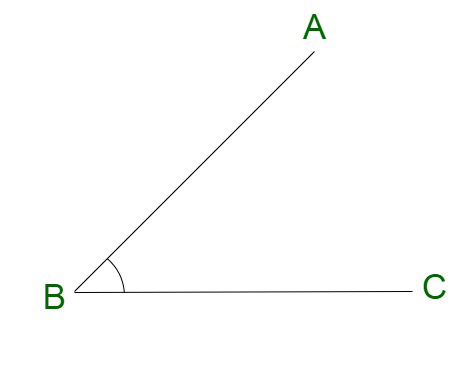Smallest Angles- Acute Angles

An acute angle is a type of angle that corresponds to a measure of less than 90°. For instance, if the time is 11 o’clock, the angle constituted between the hour hand and the minute hand of the clock is defined as an acute angle. Some of the examples are, 30°, 40°, 57°, and so on. The smallest angle is equivalent to 0o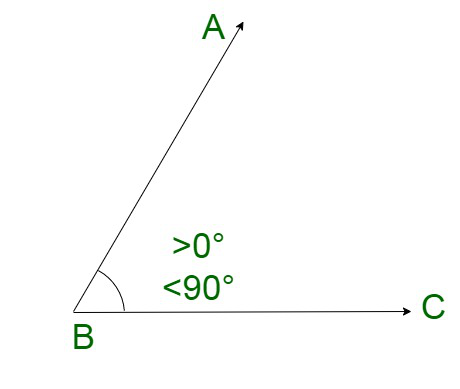Obtuse angle

Obtuse angles are generally formed on the plane surface. In Geometry, an obtuse angle is an angle that is greater than 90° and strictly less than 180°. A large number of obtuse angles are formed between a minute hand and an hour hand.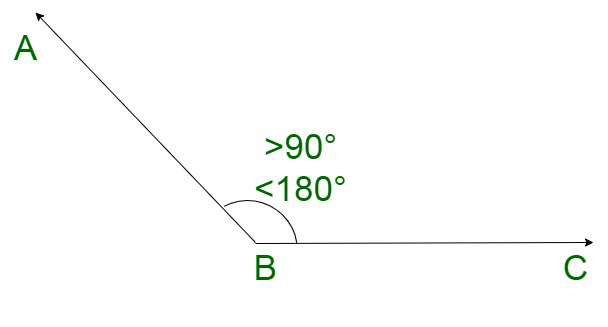Right angle

A right angle is an angle measuring 90 degrees. It is evidently visible in the corners of a room or the edges of boxes. The sides of the square and rectangle always form right angles at all the corners.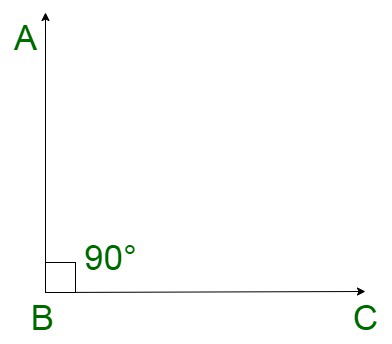Straight Angle

A straight angle corresponds to a straight line which is used to measure 180°. It is an angle whose rays are located in opposite directions from each other arising from the vertex and then, they merge together to form 180°.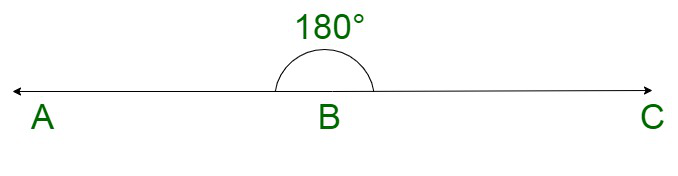Largest Angle- Reflex Angle

A reflex angle is an angle that lies between the intervals of straight angles, that is, 180° and 360°. A reflex angle and the full figure corresponding angle to form a complete angle of 360°.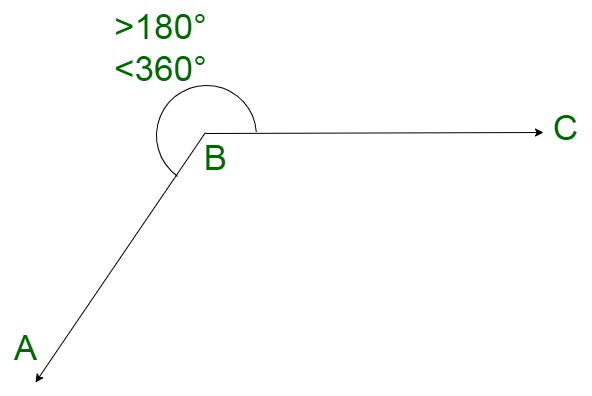### Which kind of angle is between the smallest and the largest?

Solution:

Acute angle(Smallest) < Obtuse Angle < Right Angle < Straight Angle < Reflex Angle (Largest)

### Sample Questions

Question 1. Can a triangle have two 90 degree angles?

Solution:

According to the angle sum property of triangles,

Sum of all the three angles of a triangle is equal to 180 degrees.

If there are two right angles, then the third angle will be equivalent to 0 degrees, which is not possible.

Therefore,

A triangle cannot contain two 90 degrees or right angles.

Question 2. What are the six types of angles?

Solution:

The six major types of angles are as follows:

• Acute angle
• Obtuse angle
• Right angle
• Straight angle
• Reflex angle
• Full rotation

Question 3. What is the value of an angle equal to 60 degrees, in radians?

Solution:

Since, 180 degrees form a straight angle, equal to π, therefore,

Now,

60 degrees = π/180 × 60 = π/3 (in radians)

60 degrees can be expressed as π/3 (in radians).

Question 4. What are the types of angles based on rotation?

Solution:

Angles can be majorly divided into two types based on the direction of rotation:

Positive Angles: Positive angles are measured in a counterclockwise direction from the base. They are commonly drawn from the origin in the (+x, +y) plane.

Negative Angles: Negative angles are measured in a clockwise direction from the base. They are commonly drawn from the origin in the (+x, -y) plane.

My Personal Notes arrow_drop_up